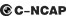## 长安 悦翔V56 种颜色可选2012款最低售价：6.19 万元起

4415(mm)1710(mm)1475(mm)##### 配置亮点：
• 胎压监测装置

• ISOFIX儿童座椅接口

• 车身稳定控制(ESC/ESP/DSC等)

• 电动天窗

• 定速巡航

• 后倒车雷达

• 真皮座椅

• GPS导航系统

• 氙气大灯

• 后视镜加热长安 悦翔V5 在售车型

排量 车型 厂商指导价 本地最低报价 购车工具
1.5L 1.5L 手动梦幻型 5挡手动 6.19万 6.19万

询底价+对比
1.5L 自动梦幻型 4挡手自一体 7.19万 7.19万

询底价+对比
1.5L 手动运动型 国IV 5挡手动 6.59万 6.59万

询底价+对比
1.5L 手动运动型 国V 5挡手动 6.69万 6.69万

询底价+对比
1.5L 自动运动型 4挡手自一体 7.59万 7.59万

询底价+对比

长安 悦翔V5 经销商

查看更多 >>
更多 >>

本地降价排名

### 长安 悦翔V5 动力加速

悦翔V5 0-100公里加速时间分布在 0.0-秒 属于 超跑级

动力级别 加速时间 车型

长安 悦翔V5 视频

长安 悦翔V5 新闻资讯

# 全新经济型小车 车展深入体验悦翔V5

评测 超过5029次关注

在本次车展上，长安汽车给我们带来了全新小型车——悦翔V5,据了解，该车是基于现款悦翔车型打造的，其定位为高品质经济性动感家轿，新车将有可能在今年下半年推出。...

# 8万元大空间自主小型车推荐 长安悦翔V5

导购 超过3731次关注

长安悦翔V5是一款基于现有悦翔车型的改款版本，悦翔是自主品牌里性价比很高的小车，便宜的价格和时尚的外形赢得了不少车主的青睐。悦翔V5在这一基础上进行了改版升...

# 源自152年前的兵工厂 忆长安汽车发展史（1）

文化 超过6744次关注

上世纪80年代，微型面包车和微型载货车红遍大江南北，“长安”的名字深深地印刻在了当时人们的脑海中。谈到国内众多的“汽车城”，重庆市可谓其中历史最为悠久的，...

# 全系下降4000元 长安悦翔V5售价调整

新闻 超过2863次关注

日前，长安汽车官方对旗下悦翔V5全系4款车型进行了售价调整，相比目前售价4款车均下降4000元，新的售价区间为6.19万-7.59万。

# 长安悦翔V5即将上市 预售价7-8万元

新闻 超过3398次关注

近日从本届成都车展长安汽车展台工作人员获悉，悦翔V5计划即将上市销售，预计售价在7-8万元。该车搭载了一台1.5升发动机。

# 长安悦翔V5正式上市 售价6.59-7.99万元

新闻 超过3168次关注

长安汽车旗下全新车型悦翔V5正式上市。悦翔V5隶属于悦翔家族，主打时尚、个性牌，定位于经济型家轿市场。

猜你喜欢

﻿
• 快速找车
• 选择品牌
• 选择品牌
• A  奥迪
• A  阿斯顿·马丁
• A  阿尔法·罗密欧
• B  宝沃
• B  布加迪
• B  巴博斯
• B  保时捷
• B  宾利
• B  奔驰
• B  宝马
• B  本田
• B  别克
• B  标致
• B  比亚迪
• B  宝骏
• B  北汽制造
• B  北汽新能源
• B  北汽幻速
• B  北汽威旺
• B  北京汽车
• B  奔腾
• B  北汽绅宝
• C  长安
• C  长安商用
• C  长城
• C  昌河
• D  大众
• D  道奇
• D  DS
• D  东南
• D  东风风神
• D  东风风行
• D  东风小康
• D  东风风度
• D  东风
• F  福特
• F  丰田
• F  菲亚特
• F  法拉利
• F  福田
• F  福迪
• F  福汽启腾
• G  观致
• G  广汽传祺
• G  广汽吉奥
• G  GMC
• H  红旗
• H  汉腾汽车
• H  哈弗
• H  哈飞
• H  海格
• H  海马
• H  华颂
• H  黄海
• H  华泰
• H  恒天
• J  吉利汽车
• J  捷豹
• J  Jeep
• J  江淮
• J  江铃
• J  金杯
• J  九龙
• J  金旅
• K  凯翼
• K  凯迪拉克
• K  克莱斯勒
• K  科尼塞克
• K  卡威
• K  开瑞
• L  路虎
• L  林肯
• L  劳斯莱斯
• L  兰博基尼
• L  雷克萨斯
• L  铃木
• L  雷诺
• L  理念
• L  力帆
• L  莲花汽车
• L  猎豹
• L  路特斯
• L  陆风
• M  马自达
• M  MG
• M  MINI
• M  玛莎拉蒂
• M  摩根
• M  迈凯轮
• N  纳智捷
• O  欧宝
• O  讴歌
• O  欧朗
• Q  奇瑞
• Q  起亚
• Q  启辰
• R  日产
• R  荣威
• R  瑞麒
• S  三菱
• S  斯威汽车
• S  萨博
• S  smart
• S  斯柯达
• S  斯巴鲁
• S  思铭
• S  双龙
• S  上汽大通
• S  双环
• T  特斯拉
• T  腾势
• W  沃尔沃
• W  五菱汽车
• W  五十铃
• W  威兹曼
• W  威麟
• X  现代
• X  雪佛兰
• X  雪铁龙
• X  西雅特
• Y  一汽
• Y  英菲尼迪
• Y  英致
• Y  依维柯
• Y  野马汽车
• Y  永源
• Z  众泰
• Z  中华
• Z  中兴
• Z  知豆
• 选择车系
• 选择车系
• 车型对比
• 选择品牌
• 选择品牌
• A  奥迪
• A  阿斯顿·马丁
• A  阿尔法·罗密欧
• B  宝沃
• B  布加迪
• B  巴博斯
• B  保时捷
• B  宾利
• B  奔驰
• B  宝马
• B  本田
• B  别克
• B  标致
• B  比亚迪
• B  宝骏
• B  北汽制造
• B  北汽新能源
• B  北汽幻速
• B  北汽威旺
• B  北京汽车
• B  奔腾
• B  北汽绅宝
• C  长安
• C  长安商用
• C  长城
• C  昌河
• D  大众
• D  道奇
• D  DS
• D  东南
• D  东风风神
• D  东风风行
• D  东风小康
• D  东风风度
• D  东风
• F  福特
• F  丰田
• F  菲亚特
• F  法拉利
• F  福田
• F  福迪
• F  福汽启腾
• G  观致
• G  广汽传祺
• G  广汽吉奥
• G  GMC
• H  红旗
• H  汉腾汽车
• H  哈弗
• H  哈飞
• H  海格
• H  海马
• H  华颂
• H  黄海
• H  华泰
• H  恒天
• J  吉利汽车
• J  捷豹
• J  Jeep
• J  江淮
• J  江铃
• J  金杯
• J  九龙
• J  金旅
• K  凯翼
• K  凯迪拉克
• K  克莱斯勒
• K  科尼塞克
• K  卡威
• K  开瑞
• L  路虎
• L  林肯
• L  劳斯莱斯
• L  兰博基尼
• L  雷克萨斯
• L  铃木
• L  雷诺
• L  理念
• L  力帆
• L  莲花汽车
• L  猎豹
• L  路特斯
• L  陆风
• M  马自达
• M  MG
• M  MINI
• M  玛莎拉蒂
• M  摩根
• M  迈凯轮
• N  纳智捷
• O  欧宝
• O  讴歌
• O  欧朗
• Q  奇瑞
• Q  起亚
• Q  启辰
• R  日产
• R  荣威
• R  瑞麒
• S  三菱
• S  斯威汽车
• S  萨博
• S  smart
• S  斯柯达
• S  斯巴鲁
• S  思铭
• S  双龙
• S  上汽大通
• S  双环
• T  特斯拉
• T  腾势
• W  沃尔沃
• W  五菱汽车
• W  五十铃
• W  威兹曼
• W  威麟
• X  现代
• X  雪佛兰
• X  雪铁龙
• X  西雅特
• Y  一汽
• Y  英菲尼迪
• Y  英致
• Y  依维柯
• Y  野马汽车
• Y  永源
• Z  众泰
• Z  中华
• Z  中兴
• Z  知豆
• 选择车系
• 选择车系
• 选择车型
• 选择车型
• 意见反馈